### Home > A2C > Chapter 12 > Lesson 12.5.2 > Problem12-208

12-208.
1. Use the values for ln 2 and ln 3 given in problem 12-207 to evaluate each of the following expressions. Do not use a calculator. Homework Help ✎

1. ln 6

2. ln12

3. ln 16

4.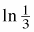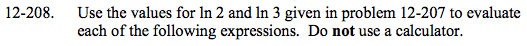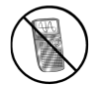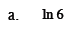$\ln6 = \ln(2 \cdot 3)$

$\ln6 = \ln2 + \ln3$

$\ln6 = (0.69315) + (1.0986)$

$\ln6 = 1.7918$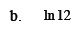See part (a).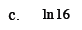See part (a).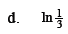$\ln\frac{1}{3} = \ln\left(3^{-1}\right)$

$\ln\frac{1}{3} = -\ln3$